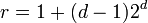# Burnside group

This article defines a group property: a property that can be evaluated to true/false for any given group, invariant under isomorphism
View a complete list of group properties
VIEW RELATED: Group property implications | Group property non-implications |Group metaproperty satisfactions | Group metaproperty dissatisfactions | Group property satisfactions | Group property dissatisfactions
This term is related to: combinatorial group theory
View other terms related to combinatorial group theory | View facts related to combinatorial group theory

## Definition

The Burnside group$B(d,n)$ (sometimes called the free Burnside group) is defined as the quotient of the free group on$d$ generators by the normal subgroup generated by all$n^{th}$ powers. A Burnside group is a group that occurs as$B(d,n)$ for some choice of$d$ and$n$.

Note that any Burnside group is a reduced free group because it is a quotient group of a free group by a verbal subgroup. More explicitly,$B(d,n)$ is free in the subvariety of the variety of groups comprising those groups where$n^{th}$ powers are equal to the identity. In particular, any Burnside group is a group in which every fully invariant subgroup is verbal.

## Relation with Burnside problem

Further information: Burnside problem

The Burnside problem is the problem of determining the conditions on$n$ under which the Burnside groups$B(d,n)$ are all finite. For some small values of$n$, the Burnside groups are all finite, whereas for large enough values of$n$, the Burnside groups are all infinite for$d > 1$.

## Particular cases

### Values of exponent

Value of$n$ What can we conclude about$B(d,n)$? Order as a function of$d,n$ Nilpotency class in terms of$d,n$ (assume$d > 0$)
0 finitely generated free group on$n$ generators infinite not nilpotent
1 trivial group, regardless of$d$ 1 0
2 elementary abelian 2-group of rank$d$ and order$2^d$$2^d$ 1
3 2-Engel group with$n$ generators, exponent three$3^{d + \binom{d}{2} + \binom{d}{3}}$ 1 if$d = 1$
2 if$d = 2$
3 if$d \ge 3$
5 if finite, same as restricted Burnside group if finite, then$5^{34}$ for$d = 2$ 12 for$d = 2$ (if finite)
17 for$d = 3$ (if finite)
6$2^s3^t$ where$s = 1 +(d - 1)3^{d + \binom{d}{2} + \binom{d}{3}}, t = r + \binom{r}{2} + \binom{r}{3}$ where$r = 1 + (d - 1)2^d$ not nilpotent

### Value pairs

Value of$n$ (we assume$n \ge 2$ to avoid the free and trivial cases) Value of$d$ (we assume$d \ge 1$ to avoid the trivial group case) Group$B(d,n)$ Order Nilpotency class
2 1 cyclic group:Z2 2 1
2 2 Klein four-group 4 1
2 3 elementary abelian group:E8 8 1
2 4 elementary abelian group:E16 16 1
3 1 cyclic group:Z3 3 1
3 2 unitriangular matrix group:UT(3,3) 27 2
3 3 Burnside group:B(3,3) 2187 3
3 4 Burnside group:B(4,3)$3^{14}$ 3
4 1 cyclic group:Z4 4 1
4 2 Burnside group:B(2,4) 4096 5
4 3 Burnside group:B(3,4)$2^{69}$  ?
4 4 Burnside group:B(4,4)$2^{422}$  ?
4 5 Burnside group:B(5,4)$2^{2728}$  ?

## Relation with other properties

### Stronger properties

Property Meaning Proof of implication Proof of strictness (reverse implication failure) Intermediate notions
Finitely generated free group Burnside group$B(d,0)$ |FULL LIST, MORE INFO

### Weaker properties

Property Meaning Proof of implication Proof of strictness (reverse implication failure) Intermediate notions
Finitely generated group